# Create Random Forest Model

## Overview

#### VIDEO TUTORIAL: Random Forest Model

The RFM approach creates a correlation vector between the training set pattern (call template) and the spectrogram. The training set pattern (call template) is applied to each of the validated recordings. In this step the template traverses each spectrogram and produces a vector of similarities for each recording (i.e. correlations between the template and sections of the spectrogram).

In the next step, Arbimon extracts 13 features from the correlation vectors of the validated recordings: mean, median, minimum, maximum, standard deviation, maximum-minimum, skewness, kurtosis, hyper-skewness, hyper-kurtosis, histogram, and cumulative frequency histogram. These 13 features along with the presence/absence data are the input to the Random Forest Models.

The goal is to train the RFM for a binary decision of presence or absence of the species call in a recording based on the feature vectors. Model performance can be accessed through the confusion matrix.

In order to run the Random Forest model Algorithm you must first Create a Training Set. After referencing the article, start the steps below to create a Random Forest job.

Tip: Random Forest Models run optimally on both Firefox and Chrome .

## Get Started

1. Click Analysis on the top navigation bar. In the left menu, click on Random Forest Models (RFM) - Create Model and then click the + button to create a model.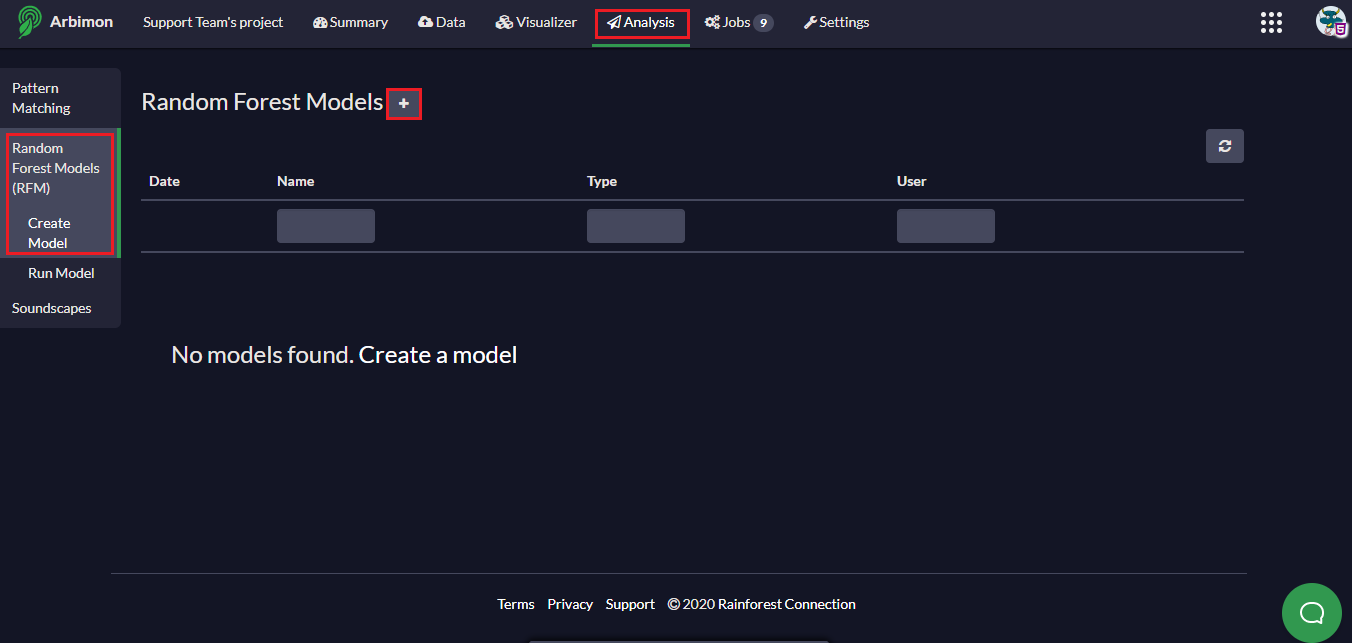2. Assign a Model Name, select Pattern Matching with Random Forests as your Classifier (default) and the Training Set (includes the template of the species call/ROIs and the validated data) from the drop menu.

The validated data is divided into two groups:

• Use in Fitting - Recordings that will be used to create the model
• Use in Validation - Recordings that will be used to validate/verify the model

One common approach in statistical modeling is to use 70-80% of validations to fit the model and 30-20% of validations to validate/verify the model. In our example, let's use 70% of your validations to fit the model and 30% to validate the model. Since presence validations are the most limited (in our example we have 234 only) let's start by applying this rule to presence validations. This means out of 234 total presence validations, we would use 163 to fit the model and 71 presence validations to verify the model. For your first model we recommend using an equal number of absence and presence recordings for the "in fitting" column, so let's include 163 absence validations to match the 163 presence validations. That means we've included 163 absence validations to fit the model and and 71 absent validations to verify/validate the model. Note that this means we are discarding 1,193 recordings with absence validations, but this is fine to do for our first model.

##### Tip:

For a correct interpretation of model statistics (i.e, accuracy and precision) an equal number of present and absent validations should ALWAYS be used in "in validation."

##### Tip:

If your model outputs too many false positive results, you can increase the number of absent validations to “Use in Fitting” to improve the model’s precision.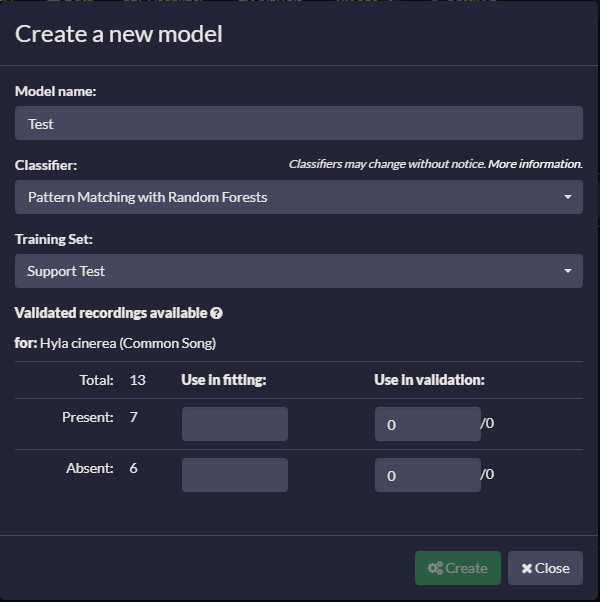3. Click Create, wait a few minutes and then click on the Refresh icon.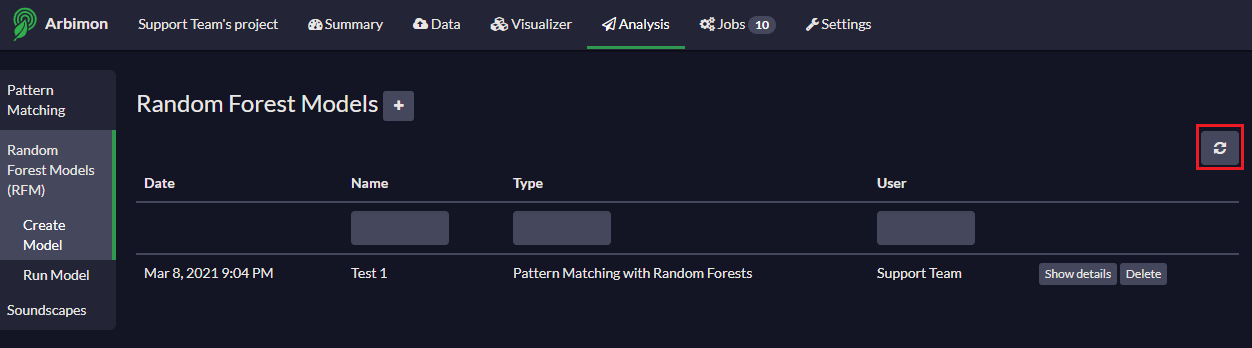4. The status of each analysis can be viewed by clicking Jobs on the top navigation bar.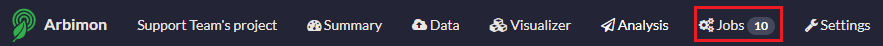5. The new model will appear in the models list. Click on Show Details to view your results.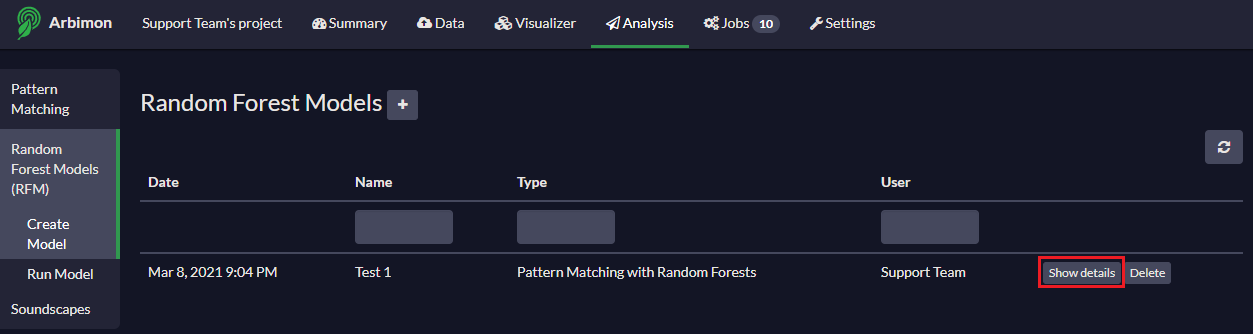Model Details shows the computed training set pattern (call template) that is used in the Random Forest Model.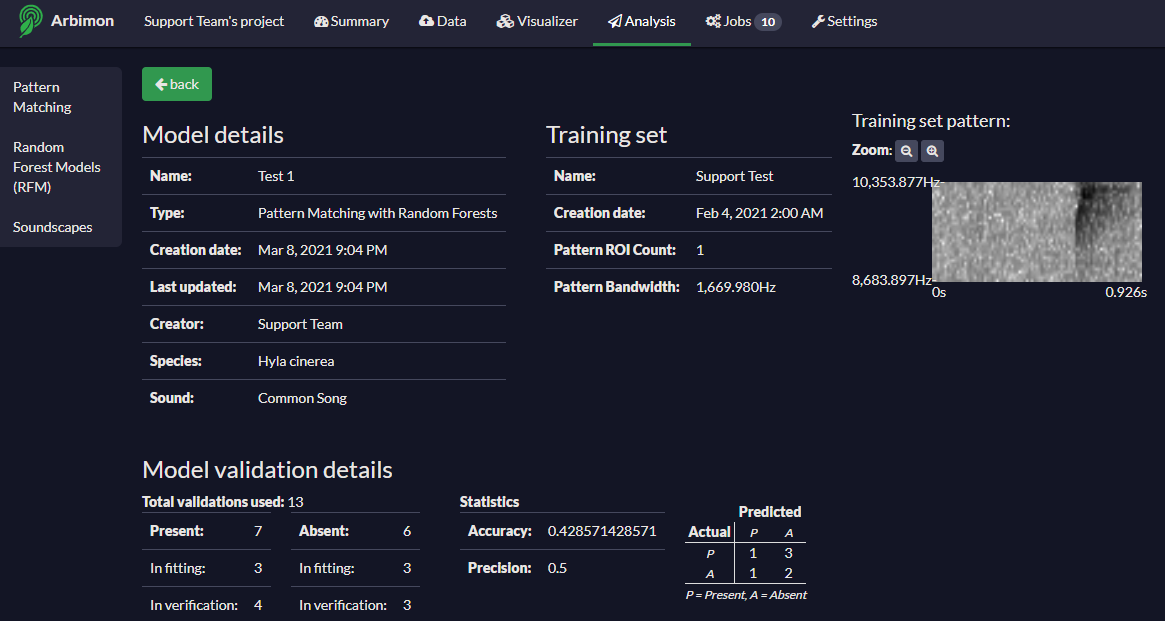Accuracy indicates overall how often your classifier is correct where TP + TN + FP + FN = total no. of validated recordings.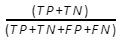Precision indicates how often your classifier is correct when it predicts that the species is present where TP + FP = is the predicted species presence.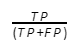The confusion matrix provides a model validation statistic describing the performance of your binary Classifier (i.e. species presence or absence). Each column of the matrix represents the number of cases or values in a predicted class, while each row represents the values in an actual class.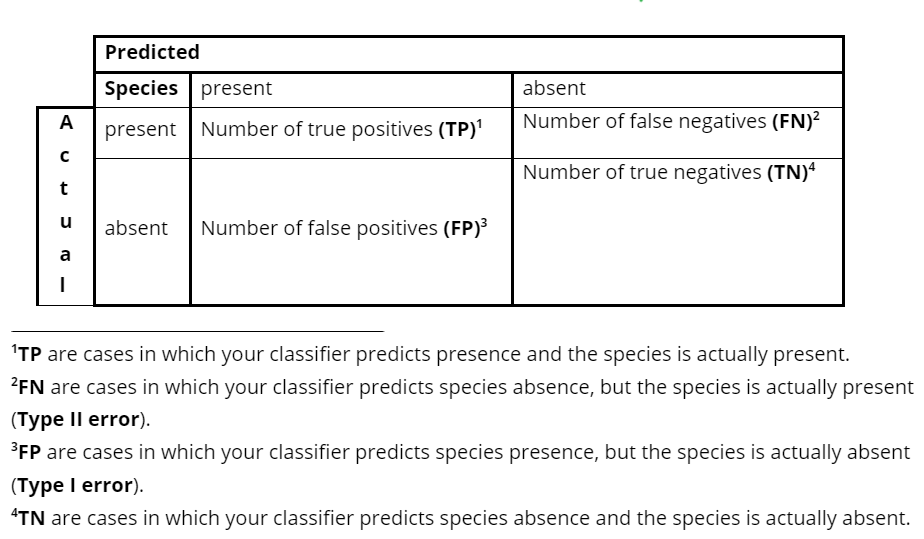Applying a threshold is an alternative approach that is based on setting manually the maximum similarity correlation level of the necessary vectors to assign a recording as having a positive detection.

New threshold - enter different values and click Recalculate to observe the changes in the confusion matrix.

##### Tip:

Try to adjust the threshold value to reduce the number of false positives.

6. Click Save Current Threshold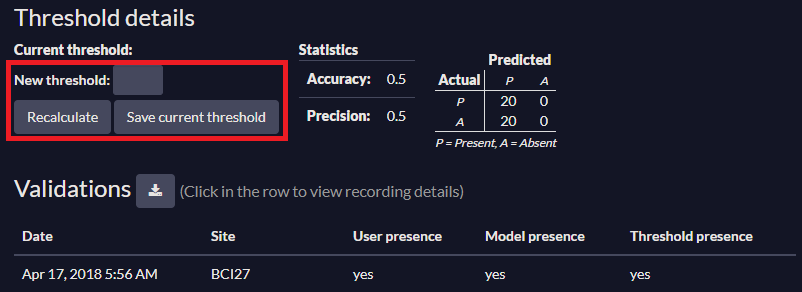7. Download results as an excel spreadsheets by clicking on the Download icon.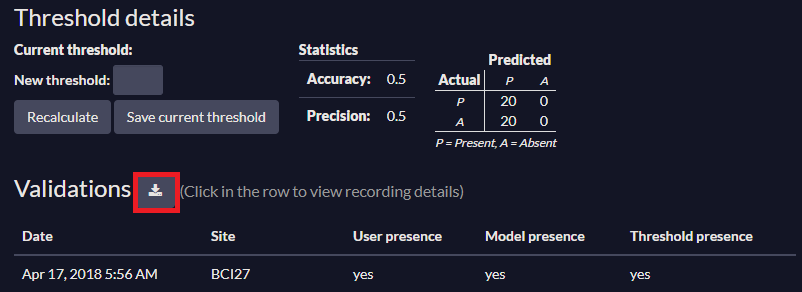Model Recommendations

• We usually want to increase the number of true positives and negatives while reducing the number of false positives and negatives.
• When evaluating the model results, the validation list below the confusion matrix allows you to explore recordings where user’s presence/absence validations did not coincide with the RF model and Threshold model approaches.

Next, you are ready to run your new RFM model over a specific playlist!